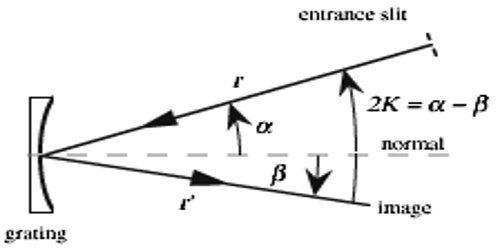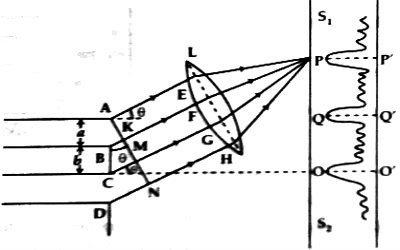# Grating Constant

Grating Constant

A diffraction grating is an optical component with a usual model. The form of the light diffracted by a grating depends on the construction of the elements. It is an optical component whose effect is alike to a prism: it splits white light into its component colors.

The distance from the starting of a slit to the starting of the next slit is called the grating constant. It is the distance between consecutive diffraction centers of an ultrasonic wave which is producing a light diffraction spectrum. It can be said in other words that, from the terminal end of a slit to the terminal end of the next slit is called the grating constant. For a diffraction grating, the grating constant is the number of lines (or slits) per unit length (eg lines per cm). Thus, the distance between the grating lines is 1/grating constant.Explanation: Suppose the width of each slit of a grating = a; and width of each line = b

According to the definition, the grating constant, d = a + b; d is sometimes called grating element.

In the length ‘d’ of the grating, number of line = 1

So, number of lines in unit length, N = 1/d

N = 1/d = 1(a+b)

Two points between the interval (a + b) of the grating is called corresponding points. In fig, A and C and B and D are a pair of corresponding points.